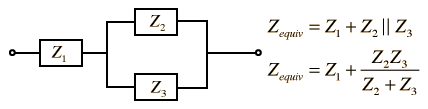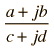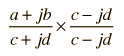# Series-Parallel Combination

Most networks in AC circuits can be broken into blocks no more complicated than the one below. The complex impedance approach provides the tool necessary to reduce such a combination to an equivalent impedance. This kind of combination may be encountered in the development of an AC Thevenin equivalent for the analysis of an AC circuit.Calculation
 The parallel part of the impedance can be put in the formwhich can be rationalized by the operation :,
and when terms are collected the results can be added to Z1 , giving the equivalent impedance.
Index

AC circuit concepts

 HyperPhysics***** Electricity and Magnetism R Nave
Go Back

# Impedance Calculation

Impedances may be combined using the complex impedance method.

For
 Z1 = + j
 Z2 = + j
 Z3 = + j

the impedance of the series-parallel combination:is Zeq = + j= at phase.

The units for all quantities are ohms. A negative phase angle implies that the impedance is capacitive, and a positive phase angle implies net inductive behavior.

 Expressions for parallel combination
 Inductive reactance Capacitive reactance
Index

AC circuit concepts

 HyperPhysics***** Electricity and Magnetism R Nave
Go Back